Related Articles
Bar Plot in Matplotlib
• Last Updated : 04 Mar, 2021

A bar plot or bar chart is a graph that represents the category of data with rectangular bars with lengths and heights that is proportional to the values which they represent. The bar plots can be plotted horizontally or vertically. A bar chart describes the comparisons between the discrete categories. One of the axis of the plot represents the specific categories being compared, while the other axis represents the measured values corresponding to those categories.

## Creating a bar plot

The matplotlib API in Python provides the bar() function which can be used in MATLAB style use or as an object-oriented API. The syntax of the bar() function to be used with the axes is as follows:-

`plt.bar(x, height, width, bottom, align)`

The function creates a bar plot bounded with a rectangle depending on the given parameters. Following is a simple example of the bar plot, which represents the number of students enrolled in different courses of an institute.

## Python3

 `import` `numpy as np``import` `matplotlib.pyplot as plt` ` ` `# creating the dataset``data ``=` `{``'C'``:``20``, ``'C++'``:``15``, ``'Java'``:``30``,``        ``'Python'``:``35``}``courses ``=` `list``(data.keys())``values ``=` `list``(data.values())`` ` `fig ``=` `plt.figure(figsize ``=` `(``10``, ``5``))` `# creating the bar plot``plt.bar(courses, values, color ``=``'maroon'``,``        ``width ``=` `0.4``)` `plt.xlabel(``"Courses offered"``)``plt.ylabel(``"No. of students enrolled"``)``plt.title(``"Students enrolled in different courses"``)``plt.show()`

Output-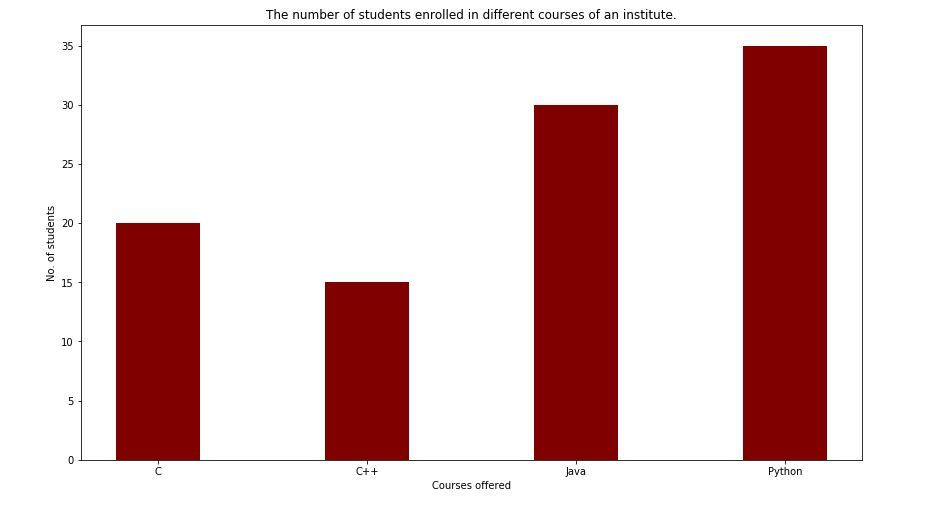Here plt.bar(courses, values, color=’maroon’) is used to specify that the bar chart is to be plotted by using the courses column as the X-axis, and the values as the Y-axis. The color attribute is used to set the color of the bars(maroon in this case).plt.xlabel(“Courses offered”) and plt.ylabel(“students enrolled”) are used to label the corresponding axes.plt.title() is used to make a title for the graph.plt.show() is used to show the graph as output using the previous commands.

## Python3

 `import` `pandas as pd``from` `matplotlib ``import` `pyplot as plt` `# Read CSV into pandas``data ``=` `pd.read_csv(r``"cars.csv"``)``data.head()``df ``=` `pd.DataFrame(data)` `name ``=` `df[``'car'``].head(``12``)``price ``=` `df[``'price'``].head(``12``)` `# Figure Size``fig ``=` `plt.figure(figsize ``=``(``10``, ``7``))` `# Horizontal Bar Plot``plt.bar(name[``0``:``10``], price[``0``:``10``])` `# Show Plot``plt.show()`

Output: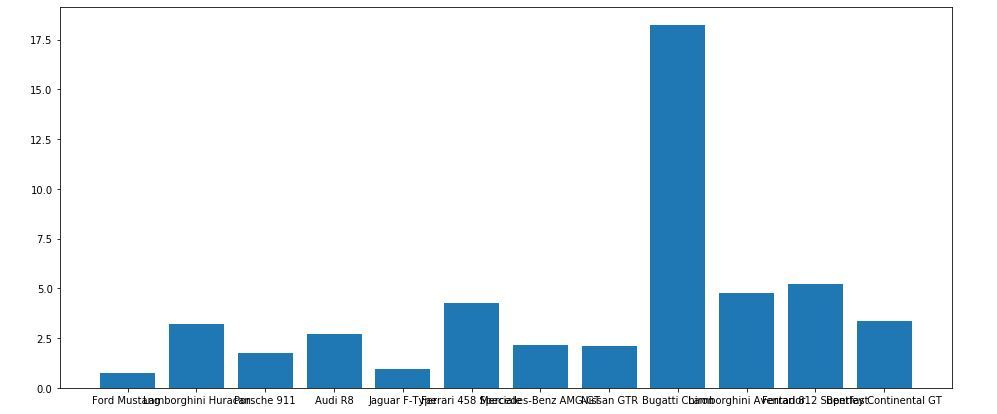It is observed in the above bar graph that the X-axis ticks are overlapping each other thus it cannot be seen properly. Thus by rotating the X-axis ticks, it can be visible clearly. That is why customization in bar graphs is required.

## Python3

 `import` `pandas as pd``from` `matplotlib ``import` `pyplot as plt` `# Read CSV into pandas``data ``=` `pd.read_csv(r``"cars.csv"``)``data.head()``df ``=` `pd.DataFrame(data)` `name ``=` `df[``'car'``].head(``12``)``price ``=` `df[``'price'``].head(``12``)` `# Figure Size``fig, ax ``=` `plt.subplots(figsize ``=``(``16``, ``9``))` `# Horizontal Bar Plot``ax.barh(name, price)` `# Remove axes splines``for` `s ``in` `[``'top'``, ``'bottom'``, ``'left'``, ``'right'``]:``    ``ax.spines[s].set_visible(``False``)` `# Remove x, y Ticks``ax.xaxis.set_ticks_position(``'none'``)``ax.yaxis.set_ticks_position(``'none'``)` `# Add padding between axes and labels``ax.xaxis.set_tick_params(pad ``=` `5``)``ax.yaxis.set_tick_params(pad ``=` `10``)` `# Add x, y gridlines``ax.grid(b ``=` `True``, color ``=``'grey'``,``        ``linestyle ``=``'-.'``, linewidth ``=` `0.5``,``        ``alpha ``=` `0.2``)` `# Show top values``ax.invert_yaxis()` `# Add annotation to bars``for` `i ``in` `ax.patches:``    ``plt.text(i.get_width()``+``0.2``, i.get_y()``+``0.5``,``             ``str``(``round``((i.get_width()), ``2``)),``             ``fontsize ``=` `10``, fontweight ``=``'bold'``,``             ``color ``=``'grey'``)` `# Add Plot Title``ax.set_title(``'Sports car and their price in crore'``,``             ``loc ``=``'left'``, )` `# Add Text watermark``fig.text(``0.9``, ``0.15``, ``'Jeeteshgavande30'``, fontsize ``=` `12``,``         ``color ``=``'grey'``, ha ``=``'right'``, va ``=``'bottom'``,``         ``alpha ``=` `0.7``)` `# Show Plot``plt.show()`

Output: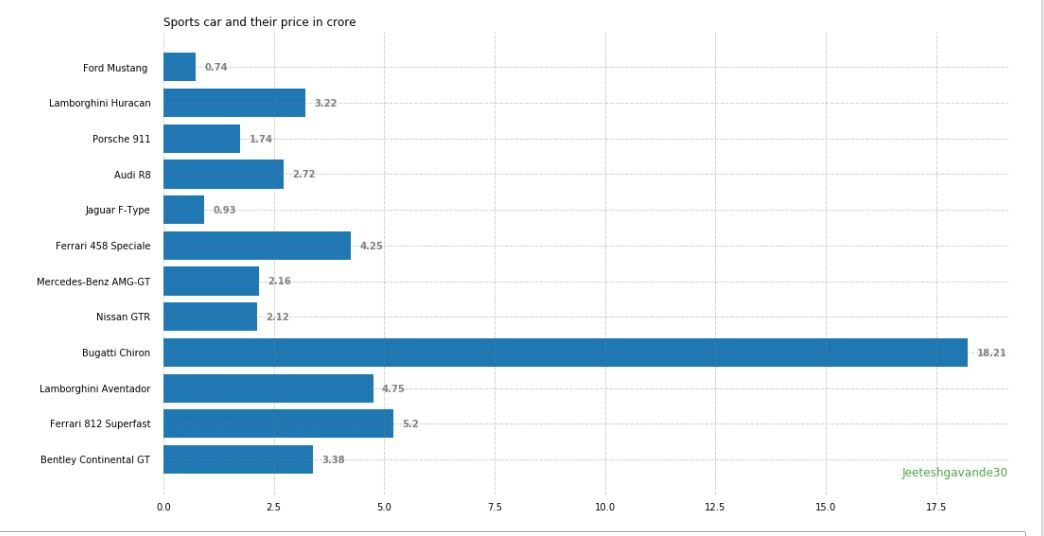There are many more Customizations available for bar plots.

## Multiple bar plots

Multiple bar plots are used when comparison among the data set is to be done when one variable is changing. We can easily convert it as a stacked area bar chart, where each subgroup is displayed by one on top of the others. It can be plotted by varying the thickness and position of the bars. Following bar plot shows the number of students passed in the engineering branch:

## Python3

 `import` `numpy as np``import` `matplotlib.pyplot as plt` `# set width of bar``barWidth ``=` `0.25``fig ``=` `plt.subplots(figsize ``=``(``12``, ``8``))` `# set height of bar``IT ``=` `[``12``, ``30``, ``1``, ``8``, ``22``]``ECE ``=` `[``28``, ``6``, ``16``, ``5``, ``10``]``CSE ``=` `[``29``, ``3``, ``24``, ``25``, ``17``]` `# Set position of bar on X axis``br1 ``=` `np.arange(``len``(IT))``br2 ``=` `[x ``+` `barWidth ``for` `x ``in` `br1]``br3 ``=` `[x ``+` `barWidth ``for` `x ``in` `br2]` `# Make the plot``plt.bar(br1, IT, color ``=``'r'``, width ``=` `barWidth,``        ``edgecolor ``=``'grey'``, label ``=``'IT'``)``plt.bar(br2, ECE, color ``=``'g'``, width ``=` `barWidth,``        ``edgecolor ``=``'grey'``, label ``=``'ECE'``)``plt.bar(br3, CSE, color ``=``'b'``, width ``=` `barWidth,``        ``edgecolor ``=``'grey'``, label ``=``'CSE'``)` `# Adding Xticks``plt.xlabel(``'Branch'``, fontweight ``=``'bold'``, fontsize ``=` `15``)``plt.ylabel(``'Students passed'``, fontweight ``=``'bold'``, fontsize ``=` `15``)``plt.xticks([r ``+` `barWidth ``for` `r ``in` `range``(``len``(IT))],``        ``[``'2015'``, ``'2016'``, ``'2017'``, ``'2018'``, ``'2019'``])` `plt.legend()``plt.show()`

Output: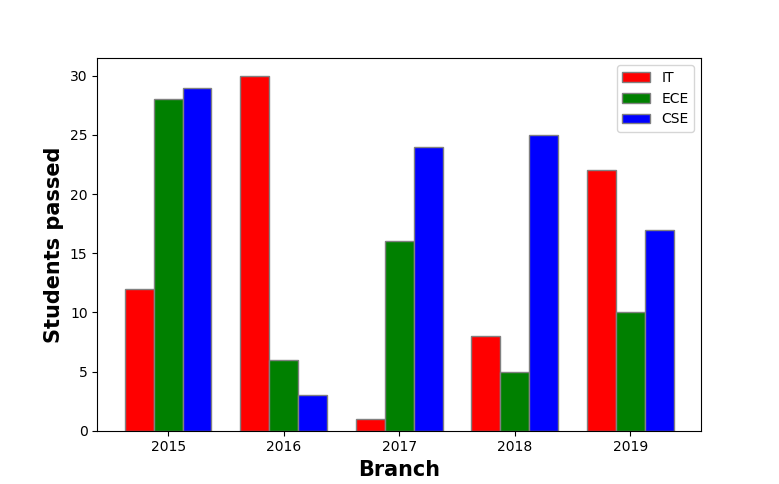## Stacked bar plot

Stacked bar plots represent different groups on top of one another. The height of the bar depends on the resulting height of the combination of the results of the groups. It goes from the bottom to the value instead of going from zero to value. The following bar plot represents the contribution of boys and girls in the team.

## Python3

 `import` `numpy as np``import` `matplotlib.pyplot as plt` `N ``=` `5` `boys ``=` `(``20``, ``35``, ``30``, ``35``, ``27``)``girls ``=` `(``25``, ``32``, ``34``, ``20``, ``25``)``boyStd ``=` `(``2``, ``3``, ``4``, ``1``, ``2``)``girlStd ``=` `(``3``, ``5``, ``2``, ``3``, ``3``)``ind ``=` `np.arange(N)  ``width ``=` `0.35`  `fig ``=` `plt.subplots(figsize ``=``(``10``, ``7``))``p1 ``=` `plt.bar(ind, boys, width, yerr ``=` `boyStd)``p2 ``=` `plt.bar(ind, girls, width,``             ``bottom ``=` `boys, yerr ``=` `girlStd)` `plt.ylabel(``'Contribution'``)``plt.title(``'Contribution by the teams'``)``plt.xticks(ind, (``'T1'``, ``'T2'``, ``'T3'``, ``'T4'``, ``'T5'``))``plt.yticks(np.arange(``0``, ``81``, ``10``))``plt.legend((p1[``0``], p2[``0``]), (``'boys'``, ``'girls'``))` `plt.show()`

Output-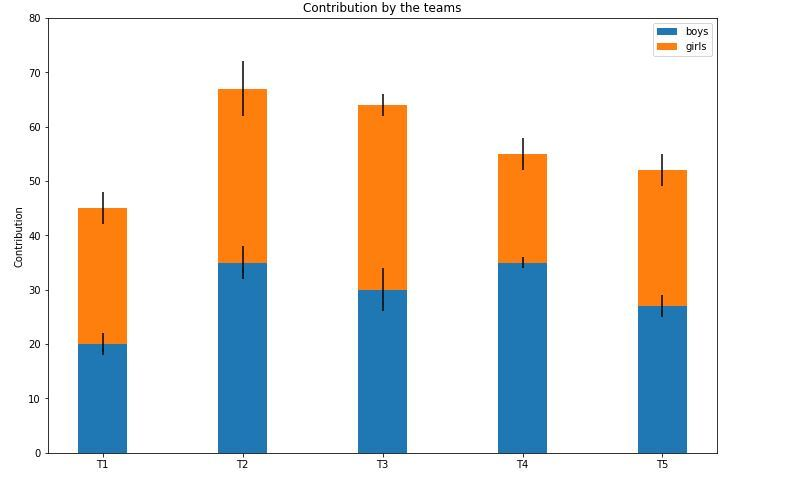Attention geek! Strengthen your foundations with the Python Programming Foundation Course and learn the basics.

To begin with, your interview preparations Enhance your Data Structures concepts with the Python DS Course.

My Personal Notes arrow_drop_up